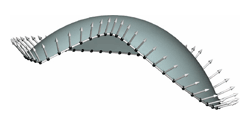Laguerre Minimal Surfaces, Isotropic Geometry and Linear Elasticity
Helmut Pottmann, Philipp Grohs, Niloy J. Mitra

Abstract:

Laguerre minimal (L-minimal) surfaces are the minimizers of the energy $\int (H^2-K)/K dA$. They are a Laguerre geometric counterpart of Willmore surfaces, the minimizers of $\int (H^2-K)dA$, which are known to be an entity of Mobius sphere geometry. The present paper provides a new and simple approach to L-minimal surfaces by showing that they appear as graphs of biharmonic functions in the isotropic model of Laguerre geometry. Therefore, L-minimal surfaces are equivalent to Airy stress surfaces of linear elasticity. In particular, there is a close relation between L-minimal surfaces of the spherical type, isotropic minimal surfaces (graphs of harmonic functions), and Euclidean minimal surfaces. This relation exhibits connections to geometrical optics. In this paper we also address and illustrate the computation of L-minimal surfaces via thin plate splines and numerical solutions of biharmonic equations. Finally, metric duality in isotropic space is used to derive an isotropic counterpart to L-minimal surfaces and certain Lie transforms of L-minimal surfaces in Euclidean space. The latter surfaces possess an optical interpretation as anticaustics of graph surfaces of biharmonic functions.

Bibtex:

@article{pgm_lag_minimal_acm_08,
AUTHOR = "Helmut Pottmann and Philipp Grohs and Niloy J. Mitra",
TITLE = "Laguerre Minimal Surfaces, Isotropic Geometry and Linear Elasticity",
JOURNAL = {Journal of Computational and Applied Mathematics},
volume={31},
number={4},
pages={391-419},
YEAR = "2008"
}